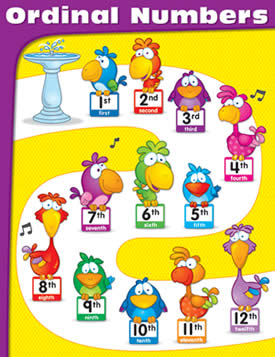# Difference between Cardinal, Ordinal and Nominal Numbers

##### Key Difference: Cardinal is used to count or indicate how many. Ordinal numbers are words that represent rank and order in a set. Nominal numbers are basically number that are used to identify something.The terms cardinal, ordinal and nominal are common terms that are used in statistics or general mathematics. The terms are used to classify numbers in a category to make it easier for use. These are often confusing for many people, but once understood it is quite simple in order to understand.

The term cardinal has two definitions: one in linguistics and one in mathematics. In linguistics or traditional grammar, the term ‘cardinal’ is a part of speech that is used to count or indicate how many. For example, a bag of 10 apples, the number 10 indicating the number of apples would be the cardinal number. Cardinal numbers are definite numerals and cannot contain fractions or decimals.However, in mathematics cardinal numbers have a slight different meaning. Cardinal refers to the measuring the cardinality (the number of elements present) of a set or between two sets. Even in this sense, cardinal numbers must have numerals or whole numbers. The cardinality of a finite set is a natural number, while the transfinite cardinal numbers describe the sizes of infinite sets. Cardinality is defined in terms of bijective functions, which requires an on-to-one function. For every element in one set, an element must be present in the pairing set. For example, Set A {1, 2, 3} and Set B {5, 6 ,7} are not equal but they have cardinality because Set A has 3 elements and so does Set B.

Ordinal numbers are words that represent rank and order in a set. The order of the set does not matter and it could in size, importance, chronology, and so on. It can also be used in English with terms such as first, second, third, etc. There are three variations to ordinal numbers: Spatial/chronological, Precedence/effect and Greek prefix. Spatial/chronological includes terms like first, second, third. Precedence/effect includes primary, secondary, tertiary, etc. Lastly, Greek prefix is the most rarely used one and uses terms such as proto-, deutero- and trito-.Nominal numbers, also known as categorical numbers are basically number that are used to identify something. The numerical value is almost the same as a name. The numerical value is irrelevant, and they do not indicate quantity, rank, or any other measurement. The usage of the term nominal is quite recent and is not broadly used. The term originates from the statistical term “nominal data” suggesting that nominal numbers are “…merely statements of qualitative category of membership.” They are often to indicate the numbers as a name. Nominal number can be categorized in two definitions "any numeral used for identification, however it was assigned", or "a numeral with no information other than identification". These numbers are not limited to using just numbers but can also include alphabets, making it alphanumerical such as license numbers, number plates on a car, etc.

Comparison between Cardinal, Ordinal and Nominal Numbers:

 Cardinal Numbers Ordinal Numbers Nominal Numbers Definition Cardinal is used to count or indicate how many. Ordinal numbers are words that represent rank and order in a set. Nominal numbers are basically number that are used to identify something. Association to remember Counting Order Name Example 6 puppies 1 child 12 cats 55 apples 1st footman 3rd of October 8th child in the family Third Saturday of the month Social security numbers Zip code Bank codes Routing numbers Car model number Other names N/A N/A Categorical

Image Courtesy: factmonster.com, mylittlepencilcase.blogspot.com, really-learn-english.com